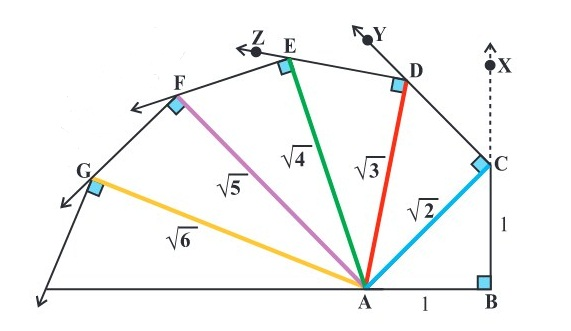# Objective: To construct a square root spiralLets see how to construct a square root spiral. Before conducting the experiment please go through and study the basic concepts of number line, concept of irrational numbers and Pythagoras theorem.

## Materials Required

2. Geometry box
4. Drawing pins
5. Nails
6. Sketch pens
7. Marker
8. A piece of plywood

### Prerequisite Knowledge

1. Concept of number line
2. Concept of irrational numbers
3. Pythagoras theorem

### Theory

A number line is a imaginary line whose each point represents a real number. The numbers which cannot be expressed in the form p/q where q ≠ 0 and both p and q are integers, are called irrational numbers, e.g. √3, π, etc. According to Pythagoras theorem, in a right angled triangle, the square of the hypotenuse is equal to the sum of the squares of other two sides containing right angle. ΔABC is a right angled triangle having right angle at B.

Therefore, AC2 = AB2 +BC2
where, AC = hypotenuse, AB = perpendicular and BC = base

### Procedure

1. Take a piece of plywood with dimensions 30 cm × 30 cm.

2. Taking 2 cm = 1 unit, draw a line segment AB of length one unit.

3. Construct a perpendicular BX at the line segment AB using set squares (or compasses).

4. From BX, cut off BC = 1 unit. Join AC.

5. Using blue coloured thread (of length equal to AC) and adhesive, fix the thread along AC.

6. With AC as base and using set squares (or compasses), draw CY perpendicular to AC.

7. From CY, cut-off CD = 1 unit and join AD.9. With AD as base and using set squares (or compasses), draw DZ perpendicular to AD.

10. From DZ, cut off DE = 1 unit and join AE.

11. Fix green coloured thread (of length equal to AE) along AE with adhesive

Repeat the above process for a sufficient number of times. This is called ?a square root spiral?.

### Demonstration

From the figure, AC2 = AB2 + BC2 = 12 + 12 = 2 or AC = √2 .

AD2= AC2 + CD2 = 2 + 1 = 3 or AD = √3

Similarly, we get the other lengths AE, AF, AG, ... as √4 or 2, √5 , √6 ...

### Observation

On actual measurement

AC = ..... , AD = ...... , AE = ...... , AF = ....... , AG = .....

√2 = AC = ............... (approx)

√3 = AD = ............... (approx)

√4 = AE = ............... (approx)

√5 = AF = ............... (approx)

### Result

A square root spiral has been constructed.

### Application

With the help of explained activity, existence of irrational numbers can be illustrated.

### Viva Voce

Question 1: Define a rational number ?
Answer: A number which can be expressed in the form of p/q, where q ≠ 0 and p, q are integers, is called a rational number.

Question 2: Define an irrational number ?
Answer: A number which cannot be expressed in the form of p/q, where q ≠ 0 and p, q are integers, is called an irrational number.

Question 3: Define a real number ?
Answer: A number which may be either rational or irrational is called a real number.

Question 4: How many rational and irrational numbers lie between any two real numbers?
Answer: There are infinite rational and irrational numbers lie between any two real numbers.

Question 5: Is it possible to represent irrational numbers on the number line?
Answer: Yes, as we know that each point on the number line represent a real number (i.e. both rational and irrational), so irrational number can be represented on number line.

Question 6: In which triangle, Pythagoras theorem is applicable?

Question 7: Give some examples of irrational numbers ?
Answer: Some examples of irrational numbers are √5, 3 ? √7, 2π, etc.

Question 8: Can we represent the reciprocal of zero on the number line?
Answer: No, because reciprocal of zero is undefined term, so we cannot represent on number line.

Question 9: In a square root spiral, is it true that in each square root of natural number is equal to the square root of the sum of 1 and previous natural number (> 1)?

Question 10: Is it possible that we make a square root spiral of negative nymbers?

### Latest Articles### Spreading Knowledge Across the World

USA - United States of America  Canada  United Kingdom  Australia  New Zealand  South America  Brazil  Portugal  Netherland  South Africa  Ethiopia  Zambia  Singapore  Malaysia  India  China  UAE - Saudi Arabia  Qatar  Oman  Kuwait  Bahrain  Dubai  Israil  England  Scotland  Norway  Ireland  Denmark  France  Spain  Poland  and  many more....